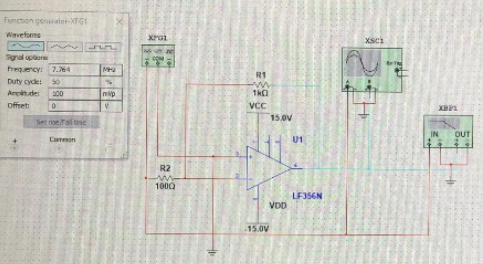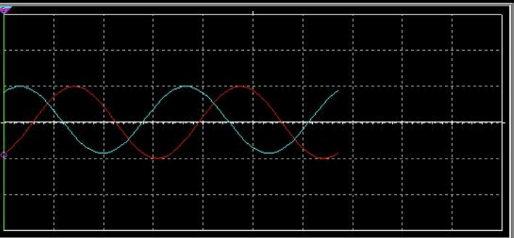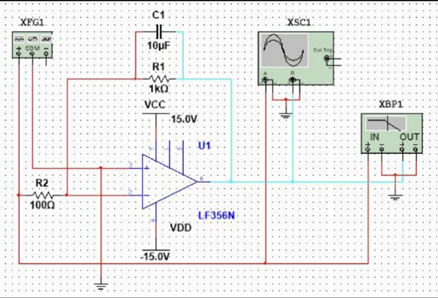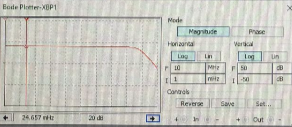Loading...

Messages

Proposals

### Guarantee Your Academic Success!

50% Off On All Assignments For New Clients. Use Coupon Code "DISCOUNT21"

# Lab Report on the Operational Amplifier

### Paper's Detail

 Category Engineering Paper Type Report Writing Reference Type APA Words 1650

The operational amplifier

Introduction of The operational amplifier

In this report, there is complete information about the operational amplifier. This report will discuss the operational amplifier in detail. In the first part of the report, there is complete information about the design calculation used for the operational amplifier. The next thing is that there is complete information about the circuit design used for this report. In the first part, the design calculation will be presented of the low pass filter and also for the wien bridge oscillator  (Classes.engineering., 2019).

Moreover, the software simulations are presented about these amplifier with their simulated waveforms. Then after this the results are tested by changing the values of resistance and capacitors. Then there is some difference between the multisim and practical results of the amplifiers. In the last, there is complete information about the frequency response graphs (Nptel. Aac.in/courses).

Design calculations of The operational amplifier

First of all there is complete design calculations for the low pass filter that is applied on the operational amplifier. For that case we just have to assume it is an ideal operational amplifier so according to that the current conversion will become

The voltage gain will become

Now rearrange the equation and also applied integration from 0 to t

From the above equation it can be noted that the output voltage is the integral of the input. On the other hand  is the voltage constant.

Now for the frequency domain analysis, it will be done through expressing the impedance of the feedback components. Due to this the transfer function can be written as

According to the information the above equation is showing that there is 90 degree phase difference that is present between the input and output signal. This shift is present at all frequencies. Due to this the gain of this amplifier will become

The feedback path is consisted of the capacitor. Through this it will become easy to calculate the equivalent impedance path

According to this the transfer function that is also the main gain of the amplifier will become

For getting the values applied the AC and DC analysis on the operational amplifier. For the DC analysis of the operational amplifier cut off the capacitors. And apply KVL on the circuit  (Brisebois, 2009).

Detail comments related to circuit design of The operational amplifier

For designing the circuit there is need to evaluate the value of capacitor and resistance. Through these values it will be simple to design this circuit. For designing the circuit there is need to apply the AC and DC analysis on the operational amplifier. According to the information the first operational amplifier is the High gain amplifier. In that particular amplifier the gain of the amplifier is extremely high. Due to this factor, we will use non inverting amplifier and its gain will be

According to the information for designing the high gain amplifier is simple. The main reason is that the value of the resistance will be adjusted at such a way so that its gain will become extremely high. According to the given scenario there is need of voltage gain of 10x.

In the next circuit, there is complete design of the low pass filter. For that case, there is need of 1 kHz frequency. But the voltage gain will be remained the same. The main thing is that the value of capacitor and resistor is adjusted in such way so that there will be no voltage loss in the circuit and required voltage gain is achieved, then after this checked the gain on the oscilloscope

And analyze the voltage gain of the system.

In the last, there is implementation of the Wien Bridge Oscillator. For that oscillator there is need of positive feedback at the output. For this the circuit is implemented in such a way that there will be positive feedback at the output of the circuit. The design of this oscillator is not too much simple. The main reason is that there is parallel and series combination of the resistor and capacitor are applied in such way for obtaining the positive feedback at the output.

Multisim simulationsThis is the circuit for the high gain amplifier. For that circuit, we have use the R1 of 1k and the R2 of 100 ohms. Through this value we are able to achieve the value of 10x gain at the required output. On the other hand the duty cycle that is applied on the input side is 50 and the required amplitude of the wave is applied at the input is 100. The input frequency of the circuit is about 7.764 Hz. The operating voltages of the operational amplifier is set at 15 volts.This is the required waveform that is giving information about the gain of the operational amplifier. This waveform is obtain by double clicking on the oscilloscope. Through this, such waveform will be obtain easily without any difficulty. From this waveform it can be seen that there is 90 degree phase shift is present between these waves. This showing that the green wave is the input wave and on the other hand the red wave is denoting the output waveform of the amplifier.This is the multisim simulation of the low pass operational amplifier. For that case, the value of capacitor is set at 10 micro farad and the value of resistor R1 is adjusted at 1k ohm and the R2 is set at the 100 ohm. The voltage gain of this amplifier is the same as the previous one, as it can be seen from this image.

Production of The operational amplifier

For the production of wave, the input voltages are set at 15 volts for both the circuits. The next thing is that the operating voltages of the operational amplifier is set at 15.6 volts.

Difference between practical and Multisim results

There is a lot of difference between the practical and software results. It can be seen that in the software simulation the results are completely absolute and perfect. This means that through the help of software results will can easily obtain the real values of the amplifier. But the next thing is when the practical is performed there is some difference in the output values.

This can be seen on the oscilloscope. There is also difference in the output voltage gain that is obtained by this amplifier. There are a lot of reason that will give complete information why there are differences are present between the voltage gain and the oscilloscope results of the amplifier. The first reason is that the value of the resistors and capacitors are not absolute. There is some difference present between these values. Due to these differences the value of the output voltages has been changed completely. This is the reason why there is somehow difference is present in the practical values.

Comments on frequency response graphs

The frequency response graphs are implemented on the log table and also on the software through the help of bode plot function.It can be noted that from the graph the horizontal frequency is adjusted at the 10 MHz and the vertical one is arranged at 50 db. By turning on the magnitude mode the frequency response is displayed on the window. This shows that the cutoff frequency is set at 20 dB and after this value the magnitude of the frequency started to going down towards the main value.

Now the work for the phase diagram of the frequency response